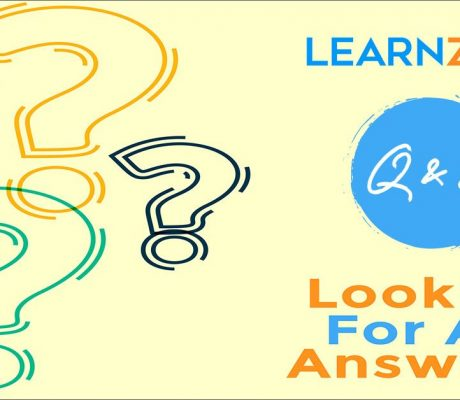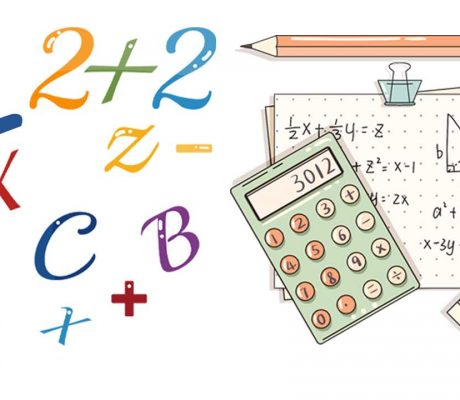# Category: Algebra### Learn ZOE Math Questions with Answers

In this blog, there are 21 math questions that Learn ZOE teachers prepared for you. The questions are based on different grade levels and once the answer is revealed, there will be a detailed explanation of how we got the correct answer. Feel free to answer the questions first before revealing the answers. Kindergarten Questions […]

Read More### What is an Equation – Math Open Reference

In mathematics, an equation is a statement that two expressions are equal. It consists of two expressions on the left and right sides of the “equals” symbol. For example, The equation states that 7 + 8 is equal to 15, which is indeed true. The most common equations include one or more variables (symbols that […]

Read More